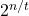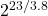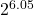## The radioisotope radon-222 has a half-life of 3.8 days. How much of a 73.9-g sample of radon- (3 222 would be left after approximately 23 da

Question

The radioisotope radon-222 has a half-life of 3.8 days. How much of a 73.9-g sample of radon- (3 222 would be left after approximately 23 days?​

in progress 0
6 months 2021-07-23T15:11:44+00:00 1 Answers 10 views 0

1.115 g

Explanation:

Applying,

R = R’………………. Equation 1

Where R = original sample of radon-222, R’ = sample of radon-222 left after decay, n = Total time, t = half-life.

make R’ the subject of the equation

R’ = R/()…………… Equation 2

From the question,

Given: R = 73.9 g, n = 23 days, t = 3.8 days.

Substitute these values into equation 2

R’ = 73.9/()

R’ = 73.9/R’ = 1.115 g